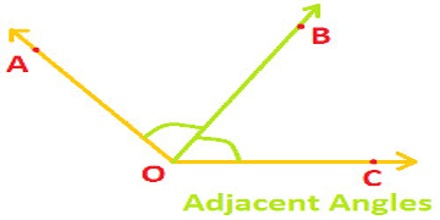Acute angle: An angle whose measure is less than 90 degrees. An angle whose measure is between 0° and 90°. The following is an acute angle.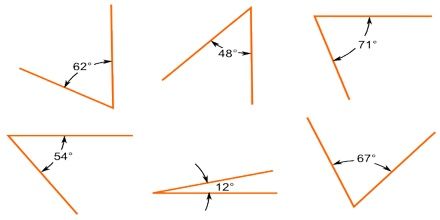Right angle: Right angles are one of the most common and interesting angles in all of geometry. It is an angle whose measure is 90 degrees. It is an internal angle which is equal to 90°. The following is a right angle.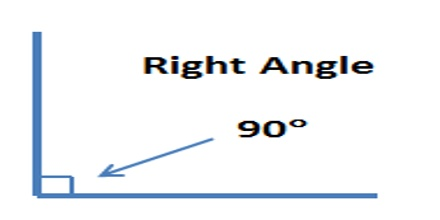Obtuse angle: An angle whose measure is bigger than 90 degrees but less than 180 degrees. Thus, it is between 90 degrees and 180 degrees. Obtuse angles are simply angles larger than 90 degrees. We can spot them because they extend past what would be a right angle. The following is an obtuse angle.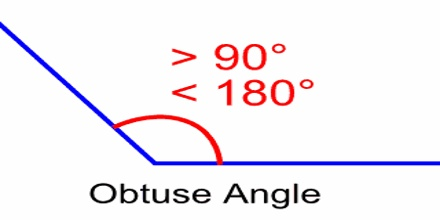Straight angle: A straight angle changes the direction to point the opposite way. It is an angle whose measure is 180 degrees. Thus, a straight angle looks like a straight line. The following is a straight angle.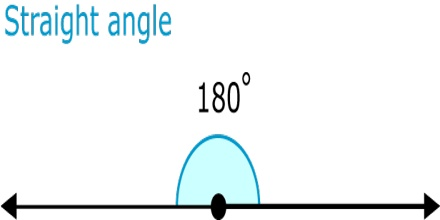Reflex angle: An angle whose measure is bigger than 180 degrees but less than 360 degrees. The measure of a reflex angle is added to an acute or obtuse angle to make a full 360 degree circle. The following is a reflex angle.Adjacent angles: Angle with a common vertex and one common side. <1 and <2, are adjacent angles.